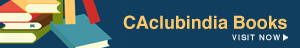FROM : CA. TEJAS K. ANDHARIA
BHAVNAGAR 364001
Phone no. 0278-6546969
Cell No. 9898709042

Beta - The Proper Understanding

Every investor wants to minimize the risk, in relation to the specified level of return. This risk is measured in the terms of beta co-efficient. Beta is the relative measure. i.e. beta of particular security or class of securities is measured in relation to the market beta. Market beta is always deemed to be 1(one). Thus, if beta of particular security is more than 1(one), it is more risky and consequently the investor would certainly want higher return and vice-versa.

Beta is actually a statistical phenomenon. If one understands its roots in the statistics, he will never make a mistake in finding and applying the beta in practical terms. Consider the following example.

 Year Return on security "A" Market return 1 25% 21% 2 28% 23% 3 31% 28%

Now we will find out the beta by applying 4(four) different formulae with proper presentation which would help you to the great extent to understand the beta efficiently.

 Year Return on security "A" (X) Market return (Y) _ (X-X) _ (Y-Y) _ 2 (X-X) _ 2 (Y-Y) _       _ (X-X) (Y-Y) 1 25 21 -3 -3 9 9 9 2 28 23 0 -1 0 1 0 3 31 28 3 4 9 16 12 Total _ X = 28 _ Y = 24 0 0 18 26 21

Formula: - 1. B = r * σx   = 0.97 * 2.45 / 2.95 = 0.81
Σy
(Here r = correlation co-efficient, σx = standard deviation of x and σy = standard deviation of y)

_         _
Where, r∑ (X-X) (Y-Y)____ = 21 / √ 18*26 = 0.97
_ 2           _ 2
√ ∑ (X-X) ∑ (Y-Y)

_ 2
σx =   √ ∑ (X-X) / n   =   √ 18 / 3 = √ 6 = 2.45

_ 2
σy =   √ ∑ (Y-Y)  / n    = √ 26 / 3 = √ 8.67 = 2.95

Formula: - 2. B = Covariance (x,y)    = ( 0.97 * 2.45 * 2.95) / (2.95 * 2.95) = 0.81
2
σy

Where, Covariance (x,y) = r * σx * σy

_         _
Formula: - 3. B = ∑ (X-X) (Y-Y)
_ 2
∑ (Y-Y)

=     21 / 26

=     0.81

Formula: - 4. This formula is related with the regression analysis that you have studied at your foundation\PE-I level in the subject of statistics.

∑ dx dy - ∑ dx dy
N
B =   ________________

∑ dy2     -   (∑ dy)2
N
Following is the regression line or characteristic line in which, if we put the value of the beta, we would get the expected behavior of return of particular security in relation to overall market risk.
_                      _
(X-X) = B *    (Y-Y)

You are advised to solve the problem by 4th formula by applying your own mind and you may refer to the study material of foundation\PE-I course to solve this.

The main point to be noted from the above exercise is – “When the value of beta is 0.81, it suggests that this security is only 81% risky in relation to the overall market risk. If it is more than 1, then it will be more risky. Market beta is always 1 and it can not be changed, as it represents the systematic risk.”

CA Tejas Andharia
(CA.)
Category Others   Report

23 Shares   6048 Views

### Popular ArticlesCCI Articles